# Logical

Library of components with Boolean input and output signals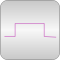# Package Contents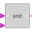And Logical 'and': y = u1 and u2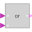Or Logical 'or': y = u1 or u2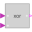Xor Logical 'xor': y = u1 xor u2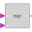Nor Logical 'nor': y = not (u1 or u2)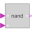Nand Logical 'nand': y = not (u1 and u2)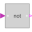Not Logical 'not': y = not u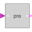Pre Breaks algebraic loops by an infinitesimal small time delay (y = pre(u): event iteration continues until u = pre(u))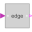Edge Output y is true, if the input u has a rising edge (y = edge(u))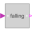FallingEdge Output y is true, if the input u has a falling edge (y = edge(not u))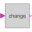Change Output y is true, if the input u has a rising or falling edge (y = change(u))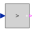GreaterThreshold Output y is true, if input u is greater than threshold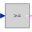GreaterEqualThreshold Output y is true, if input u is greater or equal than threshold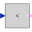LessThreshold Output y is true, if input u is less than threshold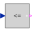LessEqualThreshold Output y is true, if input u is less or equal than threshold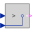Greater Output y is true, if input u1 is greater than input u2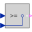GreaterEqual Output y is true, if input u1 is greater or equal than input u2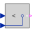Less Output y is true, if input u1 is less than input u2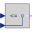LessEqual Output y is true, if input u1 is less or equal than input u2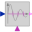ZeroCrossing Trigger zero crossing of input u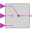LogicalSwitch Logical Switch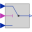Switch Switch between two Real signals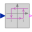Hysteresis Transform Real to Boolean signal with Hysteresis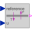OnOffController On-off controller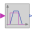TriggeredTrapezoid Triggered trapezoid generator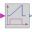Timer Timer measuring the time from the time instant where the Boolean input became true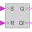RSFlipFlop A basic RS Flip Flop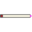TerminateSimulation Terminate simulation if condition is fulfilled

# Information

This information is part of the Modelica Standard Library maintained by the Modelica Association.

This package provides blocks with Boolean input and output signals to describe logical networks. A typical example for a logical network built with package Logical is shown in the next figure: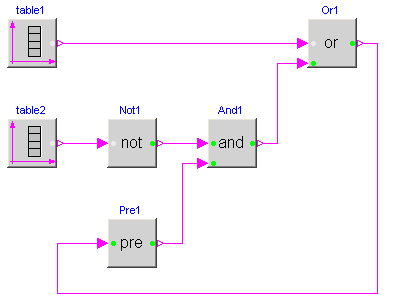The actual value of Boolean input and/or output signals is displayed in the respective block icon as "circle", where "white" color means value false and "green" color means value true. These values are visualized in a diagram animation.